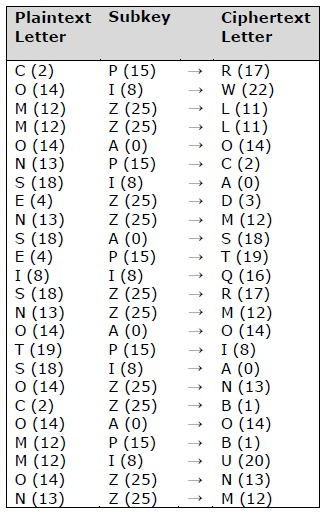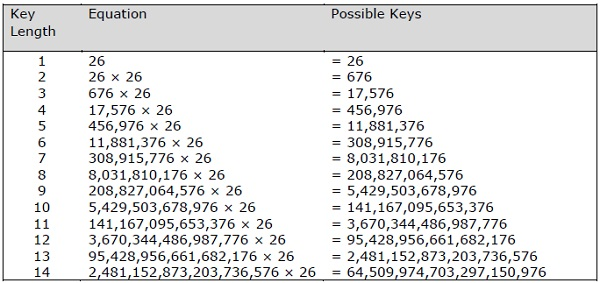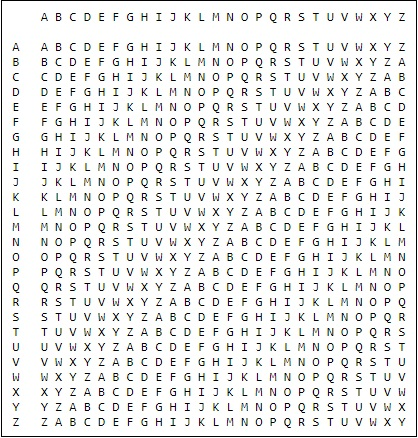# Understanding Vignere Cipher

Vignere Cipher includes a twist with Caesar Cipher algorithm used for encryption and decryption. Vignere Cipher works similar to Caesar Cipher algorithm with only one major distinction: Caesar Cipher includes algorithm for one-character shift, whereas Vignere Cipher includes key with multiple alphabets shift.

## Mathematical Equation

For encryption the mathematical equation is as follows −

$$E_{k}\left ( M{_{i{}}} \right ) = \left ( M_{i}+K_{i} \right )\;\;\; mod \;\; 26$$

For decryption the mathematical equation is as follows −

$$D_{k}\left ( C{_{i{}}} \right ) = \left ( C_{i}-K_{i} \right )\;\;\; mod \;\; 26$$

Vignere cipher uses more than one set of substitutions, and hence it is also referred as polyalphabetic cipher. Vignere Cipher will use a letter key instead of a numeric key representation: Letter A will be used for key 0, letter B for key 1 and so on. Numbers of the letters before and after encryption process is shown below −The possible combination of number of possible keys based on Vignere key length is given as follows, which gives the result of how secure is Vignere Cipher Algorithm −## Vignere Tableau

The tableau used for Vignere cipher is as shown below −